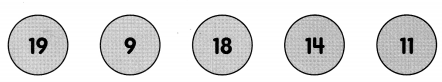# Math in Focus Grade 1 Chapter 7 Practice 3 Answer Key Comparing Numbers

This handy Math in Focus Grade 1 Workbook Answer Key Chapter 7 Practice 3 Comparing Numbers detailed solutions for the textbook questions.

## Math in Focus Grade 1 Chapter 7 Practice 3 Answer Key Comparing Numbers

Write the number in each set. Then fill in the blanks.

Example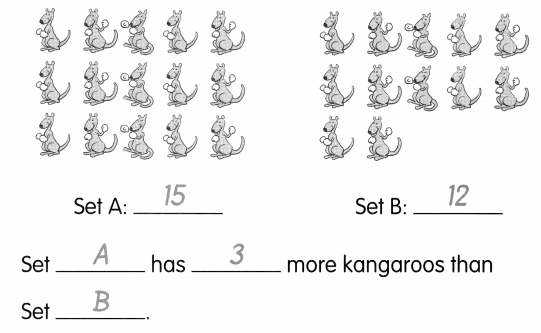Question 2.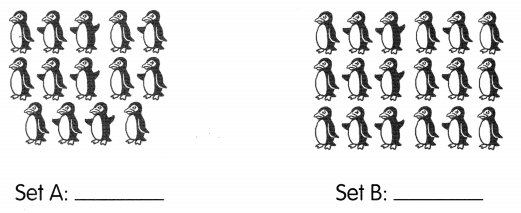Set ___ has ___ more penguins than Set ____
Set A: 14
Set B: 18
Set B has 4 more penguins than Set A.

Write the number in each set. Then fill in the blanks.

Question 2.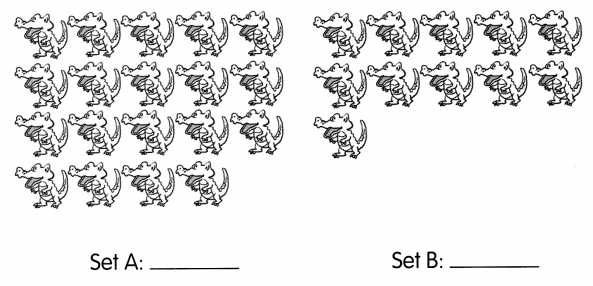Set ___ has ___ more crocodiles than Set ____
Set A: 19
Set B: 11
Set A has 8 more crocodiles than Set B

Question 3.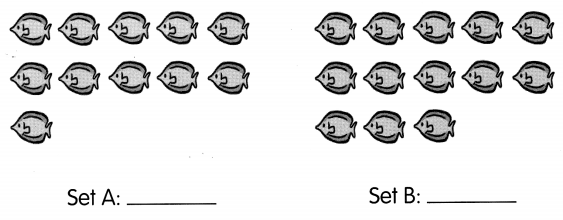Set ___ has ___ fewer fish than Set ___
Set A: 11
Set B: 13
Set A has 2 fewer fish than Set B.

Question 4.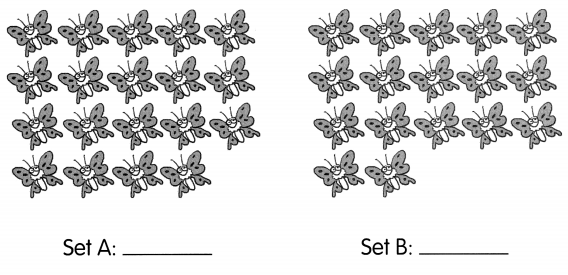Set ___ has ___ fewer butterflies than Set ___
Set A: 19
Set B: 17
Set B has 2 fewer butterflies than Set A

Question 5.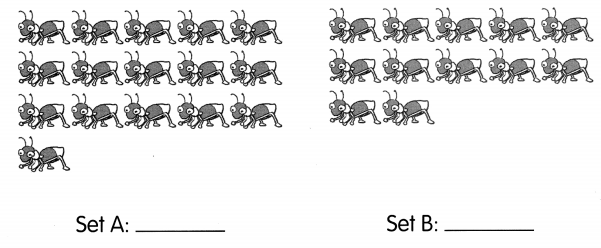Set ___ has ___ fewer ants than Set ___
Set A: 16
Set B: 12
Set B has 4 fewer ants than Set A

Color the house with the number that is less. Then fill in the blanks.

Example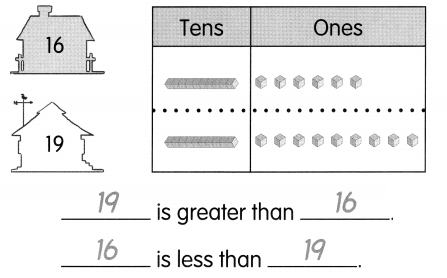Question 6.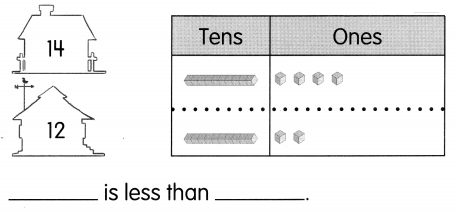Answer: 12 is less than 14

The tens are equal.
Compare the ones.
9 ones is greater than 6 ones.
6 ones is less than 9 ones.

Question 7.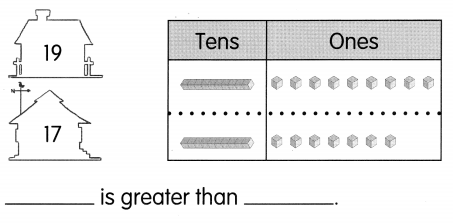Answer: 19 is greater than 17

Find the number that is less. Color the animal red. Find the number that is greater. Color the animal blue.

Question 8.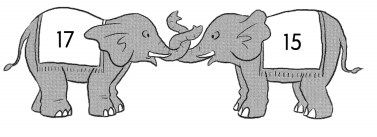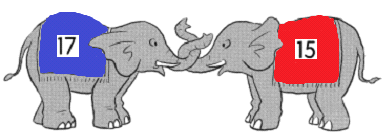Question 9.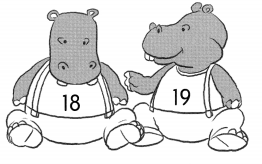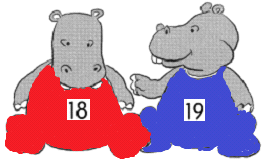Color the creature with the correct number. Then fill in the blanks.

Question 10.
the number that is greater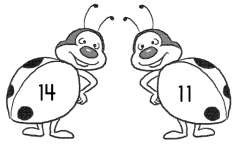How much greater is the number? _____
Answer: 14 is the greater number than 11.

Question 11.
the number that is less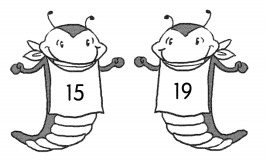How much less is the number? ____
Answer: 15 is the less number than 19.

Fill in the blanks in each place-value chart. Then color the sign with the greatest number.

Question 12.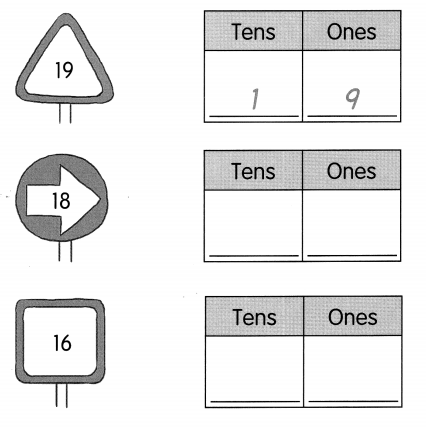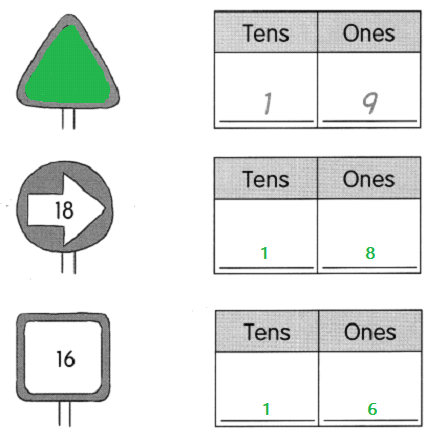Fill in the blanks in each place-value chart. Then color the sign with the least number.

Question 13.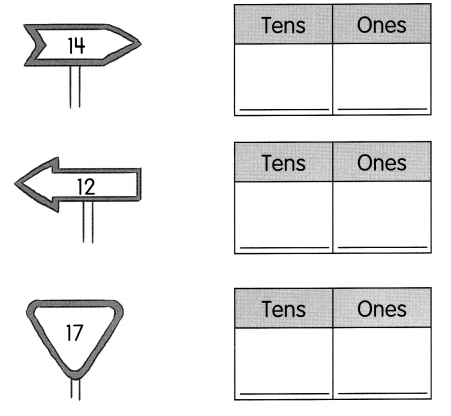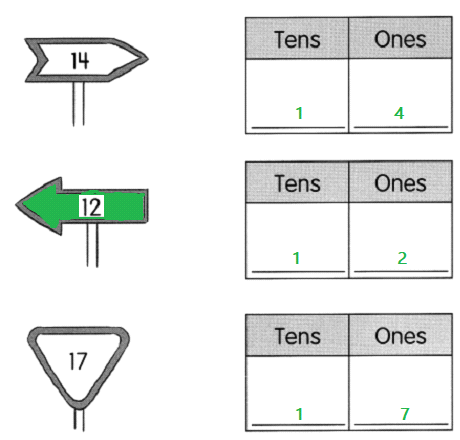Compare the numbers. Fill in the blanks.

Question 14.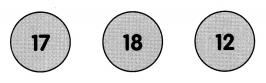____ is the least number.
_____ is the greatest number.
12 is the least number.
18 is the greatest number.

Compare the numbers.
Fill in the blanks.

Question 15.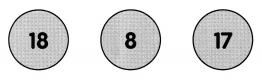___ is the least number.
_____ is the greatest number.
8 is the least number.
18 is the greatest number.

Question 16.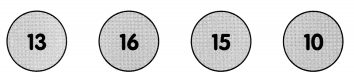____ is the least number.
____ is the greatest number.